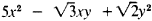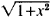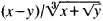# Algebraic Function

(redirected from Algebraic functions)
Also found in: Dictionary.

## algebraic function

[¦al·jə¦brā·ik ′fəŋk·shən]
(mathematics)
A function whose value is obtained by performing only the following operations to its argument: addition, subtraction, multiplication, division, raising to a rational power.
McGraw-Hill Dictionary of Scientific & Technical Terms, 6E, Copyright © 2003 by The McGraw-Hill Companies, Inc.
The following article is from The Great Soviet Encyclopedia (1979). It might be outdated or ideologically biased.

## Algebraic Function

a function that satisfies an algebraic equation; one of the most important functions studied in mathematics. Among the algebraic functions, polynomials and quotient polynomials—for example,and (1 + x + x2)/(2 + x3)—are called rational and all the rest are called irrational. The simplest examples of the latter are algebraic functions expressed by means of radicals—for example,andHowever, there are certain algebraic functions that cannot be expressed with radicals—the function y = f (x) which satisfies the equation y5 + 5yx4 + 5x5 = 0. Examples of nonalgebraic, or so-called transcendental functions, encountered in school algebra courses, are the power function xα, if α is an irrational number; the exponential function ax; the logarithmic function; and so forth. The general theory of algebraic functions is an extensive mathematical discipline having important connections with the theory of analytic functions (of which algebraic functions constitute a special class), algebra, and algebraic geometry. The most general algebraic function of many variables, u = f(x, y, z., ...), is defined as a function satisfying an equation of the type

P0(x, y, z, . . . )un + P1 (x, y, z, . . .)un−1

(1) + . . . + Pn (x, y, z, . . . ) = 0

where P0,P1,. . . Pn are any polynomials with respect to x, y, z, . . . . The entire expression in the left member represents a certain polynomial with respect to x, y, z, . . . and u. It may be considered irreducible—that is, not factorable into polynomials of lower degree; in addition, polynomial P0 can be considered as not identically equal to zero. If n = 1, then u represents a rational function (u = −P1/P0), a partial case of which—the integral rational function—is the polynomial (if P0 = const ≠ 0). When n > 1, we have an irrational function; when n = 2, the function is expressed in terms of polynomials with the use of a square root; when n = 3 or 4, for u we have an expression containing both square and cube roots.

When n ≥ 5, the irrational function u can no longer be expressed in the general case, in terms of a finite number of any roots of polynomials. An irrational algebraic function is always many-valued; it is, precisely, for our given designations and assumptions, an n-valued analytic function of variables x, y, z,.....

### REFERENCE

Chebotarev, N. G. Teoriia algebraicheskikh funktsii. Moscow-Leningrad, 1948.
References in periodicals archive ?
There is dedicated code, for example, to handle elliptic integrals and algebraic functions or special functions where specific algorithms (based on the theory of Hypergeometric PFQ and MeijerG functions) are used, .
The problems were selected from a group of more than 50 integrals tested on the three CAS; those selected cover a range of combinations of transcendental and algebraic functions and pose some difficulties to one or more of the CAS tested.
In the present subsection we define an algebraic functions [psi] of third degree together with its associated Riemann surface R in a first step, which then is followed by the definition of an harmonic function h, which will be an important element of the asymptotic relations for the rescaled Hermite-Pade polynomials [P.sub.n], [Q.sub.n], [R.sub.n] and the remainder term [E.sub.n].
Artin works through the theory of valuation, local class field theory, the elements of algebraic number theory and the theory of algebraic function fields of one variable.
Each element, or more properly, cell, is described as a high-level algebraic function. In fact, the real efficiency of BEM was lost if an engineer attempted to "overmesh" by applying a FEM meshing strategy.
Since then, there has been much use of algebraic functions in combinatorics, see e.g.
A transition chapter deals with areas such as the limit of a function and methods for computing limits of algebraic functions. Later chapters cover continuity of functions, derivatives of functions, trigonometric limits, and exponential and logarithmic functions and their derivatives, in addition to implicit functions, parametric functions, Rolle's theorem, Taylor's formula, and hyperbolic functions.
(The explanation of why such a situation occurs systematically was given in , for the case of differential equations satisfied by algebraic functions.) A good heuristic is to compute several non-minimal operators and to take their greatest common right divisor; generically, the result is exactly [L.sub.min].
First we have to obtain a closed form for the generating function [B.sup.[*](x, y, w) of rooted 2-connected planar graphs, where x marks vertices, y edges, and w the degree of the root: the main problem we encounter here is solving a differential equation involving algebraic functions and other functions defined implicitly.
Since F is rational, these singularities are poles and algebraic functions of x, so that the residue sum, the diagonal generating function, is also an algebraic function of x.

Site: Follow: Share:
Open / Close